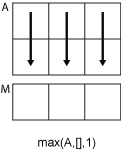# max

## 语法

``M = max(A)``
``M = max(A,[],dim)``
``M = max(A,[],nanflag)``
``M = max(A,[],dim,nanflag)``
``[M,I] = max(___)``
``M = max(A,[],'all')``
``M = max(A,[],vecdim)``
``M = max(A,[],'all',nanflag)``
``M = max(A,[],vecdim,nanflag)``
``[M,I] = max(A,[],___,'linear')``
``C = max(A,B)``
``C = max(A,B,nanflag)``

## 说明

````M = max(A)` 返回数组的最大元素。 如果 `A` 是向量，则 `max(A)` 返回 `A` 的最大值。如果 `A` 为矩阵，则 `max(A)` 是包含每一列的最大值的行向量。如果 `A` 是多维数组，则 `max(A)` 沿大小不等于 `1` 的第一个数组维度计算，并将这些元素视为向量。此维度的大小将变为 `1`，而所有其他维度的大小保持不变。如果 `A` 是第一个维度长度为零的空数组，则 `max(A)` 返回与 `A` 大小相同的空数组。 ```

``M = max(A,[],dim)` 返回维度 `dim` 上的最大元素。例如，如果 `A` 为矩阵，则 `max(A,[],2)` 是包含每一行的最大值的列向量。`

``M = max(A,[],nanflag)` 指定在计算中包括还是忽略 `NaN` 值。例如，`max(A,[],'includenan')` 包括 `A` 中的所有 `NaN` 值，而 `max(A,[],'omitnan')` 忽略这些值。`
``M = max(A,[],dim,nanflag)` 还指定使用 `nanflag` 选项时的运算维度。`

``[M,I] = max(___)` 在上述语法基础上，还返回 `A` 中最大值在运算维度上的对应索引。`

``M = max(A,[],'all')` 查找 `A` 的所有元素的最大值。此语法适用于 MATLAB® R2018b 及更高版本。`

``M = max(A,[],vecdim)` 计算向量 `vecdim` 所指定的维度上的最大值。例如，如果 `A` 是矩阵，则 `max(A,[],[1 2])` 计算 `A` 中所有元素的最大值，因为矩阵的每个元素都包含在由维度 1 和 2 定义的数组切片中。`
``M = max(A,[],'all',nanflag)` 计算在使用 `nanflag` 选项时 `A` 的所有元素的最大值。`
``M = max(A,[],vecdim,nanflag)` 指定在使用 `nanflag` 选项时要运算的多个维度。`

``[M,I] = max(A,[],___,'linear')` 返回 `A` 中最大值在 `A` 中的对应线性索引。`

``C = max(A,B)` 返回从 `A` 或 `B` 中提取的最大元素的数组。`
``C = max(A,B,nanflag)` 还指定如何处理 `NaN` 值。`

## 示例

```A = [23 42 37 18 52]; M = max(A)```
```M = 52 ```

```A = [-2+2i 4+i -1-3i]; max(A)```
```ans = 4.0000 + 1.0000i ```

`A = [2 8 4; 7 3 9]`
```A = 2×3 2 8 4 7 3 9 ```
`M = max(A)`
```M = 1×3 7 8 9 ```

`A = [1.7 1.2 1.5; 1.3 1.6 1.99]`
```A = 2×3 1.7000 1.2000 1.5000 1.3000 1.6000 1.9900 ```
`M = max(A,[],2)`
```M = 2×1 1.7000 1.9900 ```

```A = [1.77 -0.005 3.98 -2.95 NaN 0.34 NaN 0.19]; M = max(A,[],'omitnan')```
```M = 3.9800 ```

`M = max(A,[],'includenan')`
```M = NaN ```

`A = [1 9 -2; 8 4 -5]`
```A = 2×3 1 9 -2 8 4 -5 ```
`[M,I] = max(A)`
```M = 1×3 8 9 -2 ```
```I = 1×3 2 1 1 ```

```A(:,:,1) = [2 4; -2 1]; A(:,:,2) = [9 13; -5 7]; A(:,:,3) = [4 4; 8 -3]; M1 = max(A,[],[1 2])```
```M1 = M1(:,:,1) = 4 M1(:,:,2) = 13 M1(:,:,3) = 8 ```

`M2 = max(A,[],[1 2 3])`
```M2 = 13 ```
`Mall = max(A,[],'all')`
```Mall = 13 ```

`A = [1 2 3; 4 5 6]`
```A = 2×3 1 2 3 4 5 6 ```
`[M,I] = max(A,[],2,'linear')`
```M = 2×1 3 6 ```
```I = 2×1 5 6 ```
`maxvals = A(I)`
```maxvals = 2×1 3 6 ```

`A = [1 7 3; 6 2 9]`
```A = 2×3 1 7 3 6 2 9 ```
```B = 5; C = max(A,B)```
```C = 2×3 5 7 5 6 5 9 ```

## 输入参数

• 如果 `A` 是复矩阵，则 `max(A)` 返回幅值最大的复数。如果幅值相等，则 `max(A)` 返回具有最大幅值和最大相位角的值。

• 如果 `A` 是标量，则 `max(A)` 返回 `A`

• 如果 `A` 为 0×0 空数组，则 `max(A)` 也是空数组。

• 如果 `dim = 1`，则 `max(A,[],1)` 返回包含每列中的最大元素的行向量。• 如果 `dim = 2`，则 `max(A,[],2)` 返回包含每行中的最大元素的列向量。• `A``B` 的数据类型必须相同，除非其中一个是 `double`。如果是那样，另一个数组的数据类型可以是 `single``duration` 或任何整数类型。

• 如果 `A``B` 均为有序 `categorical` 数组，则必须具有顺序相同的同一类别集。

`NaN` 条件，指定为下列值之一：

• `'omitnan'` - 忽略输入中的所有 `NaN` 值。如果所有元素均为 `NaN`，则 `max` 返回第一个元素。

• `'includenan'` - 在计算输入中包括 `NaN` 值。

## 输出参数

`A``B` 中的最大元素，以标量、向量、矩阵或多维数组的形式返回。`C` 的大小由 `A``B` 的维度的隐式扩展决定。有关详细信息，请参阅基本运算的兼容数组大小

`C` 的数据类型取决于 `A``B` 的数据类型：

• 如果 `A``B` 是相同的数据类型，则 `C``A``B` 的数据类型匹配。

• 如果 `A``B``single`，则 `C``single`

• 如果 `A``B` 为整数数据类型且另一个为标量 `double`，则 `C` 采用整数数据类型。